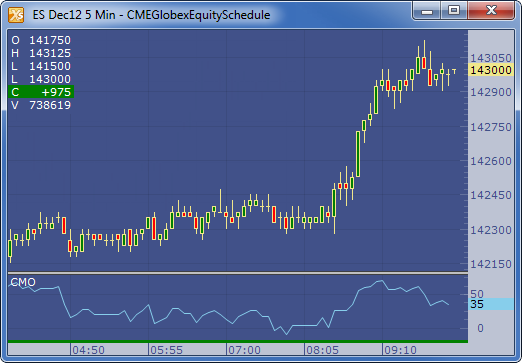← Back to X_TRADER® Help Library

### X_STUDY® Documentation

#### Chande Momentum Oscillator (CMO)

##### Description

The Chande Momentum Oscillator (CMO) developed by Tushar Chande attempts to capture the momentum of the instrument. The indicator oscillates between -100 and 100 with overbought level of 50 and oversold level of -50.

##### Formula

CMO = ((PosSum - NegSum) / (PosSum + NegSum)) * 100

Where:

• PosSum = Sum of (Closecurrent- ClosePrevious) where (Closecurrent- ClosePrevious) is greater than zero
• NegSum = Absolute value of the sum of (Closecurrent- ClosePrevious) where (Close current- Close Previous) is less than zero
##### Example